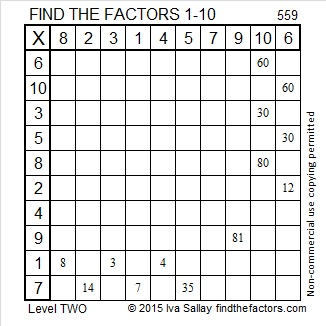671 is the Magic Sum of an 11 x 11 Magic Square

6 – 7 + 1 = 0 so 671 is divisible by 11.

671 is the sum of the fifteen prime numbers from 17 to 73.

Because 61 is one of its factors, 671 is the hypotenuse of the Pythagorean triple 121-660-671. The greatest common factor of those three numbers practically jumps out at me. Does it do the same thing to you?

Best of all 671 is the magic sum of an 11 x 11 magic square. (That link from wikipedia helped me construct this square. I’ll give directions so you can do it, too!)Notice how every row, column, and diagonal on the square sums to 671. The reason it is the magic sum is because the sum of all the numbers from 1 to 121 can be computed and then divided by 11 (the number of rows). Here is the equation:

• 671 = 121 x 120/2/11

Because 11 is an odd number there are simple directions to complete the entire square:

The number 1 is located in the exact center of the top row.

Find the number 2 on the square. (It’s located on the bottom row just right of the exact center square.) Notice that the numbers 3, 4, 5, and 6 are on the same diagonal. If you imagine the diagonal wrapping around the square, you can continue to follow it for numbers 7, 8, 9, 10, and 11. We can’t put the number 12 along the same diagonal because the number 1 is already in that spot, so we put the 12 UNDER the 11 and begin working on a new diagonal.

Anytime a number already occupies a space on a diagonal, put the next number under the preceding number and continue making a new diagonal. When a diagonal reaches the edge of the square, imagine that edge is connected to the opposite edge and continue the diagonal from the opposite edge.

I found it to be the trickiest placing the numbers 67 and 68, but other than that it was rather easy to know where to put the numbers.

Notice that the difference between any smaller number and the larger number just below it is either 12 or 1.

If you have excel on your computer, click on 12 Factors 2015-11-02, select the magic square tab, and then you can make this 11 x 11 magic square yourself. As you type in numbers, the columns, rows, and diagonals will automatically keep a running sum.

Once you get the square to give the magic sum in each direction, you can try doing the same thing with the 13 x 13 magic square that I’ve included on the same page. Its magic sum is 1105 which can be also be computed:

• 1105 = 169 x 170/22/13.

There is actually many more possible and probably more complicated 11 x 11 and 13 x 13 magic squares. I hope you enjoy making some with this easy method.

—————————————————————————————————

• 671 is a composite number.
• Prime factorization: 671 = 11 x 61
• The exponents in the prime factorization are 1 and 1. Adding one to each and multiplying we get (1 + 1)(1 + 1) = 2 x 2 = 4. Therefore 671 has exactly 4 factors.
• Factors of 671: 1, 11, 61, 671
• Factor pairs: 671 = 1 x 671 or 11 x 61
• 671 has no square factors that allow its square root to be simplified. √671 ≈ 25.903668.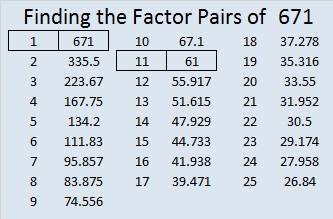—————————————————————————————————

What often happens to a number like 599 when a number next to it has so many factors?

The square root of any number from 576 to 624 is between 24 and 25. That means the first number in any of their factor pairs will be 24 or less. The combined number of factor pairs for the 49 integers from 576 to 624 is 189. The number of factor pairs for any given number ranges from 1 to 12. Let’s look at the averages: The mean (189/49) is 3.857 factor pairs per number. The median is 3 factor pairs, and the mode (the number of factor pairs that occurs most often) is 4 factor pairs.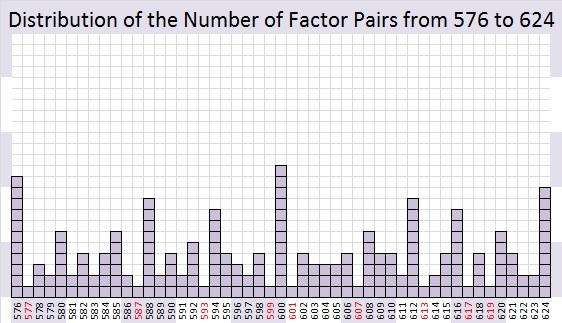What happens to a number like 599 when a number next to it has far more than the average number of factor pairs? Quite often, but not always, that number has no choice but to be a prime number.Even though 600 has only three prime factors (2, 3 and 5), it still managed to be divisible by 50% of the numbers from 1 to 24, and there just aren’t many possibilities left for the numbers immediately before or after it.

The twelve numbers less than or equal to 24 that will divide into 600 are 1, 2, 3, 4, 5, 6, 8, 10, 12, 15, 20 and 24.

Because 9, 14, 16, 18, 21, and 22 each have 2 or 3 as a prime factor, those six numbers also are not possible factors of 599 or 601.

Every whole number is divisible by 1, but besides that, there are only six numbers available as possible factors for those two numbers: 7, 11, 13, 17, 19, and 23. Since neither 599 nor 601 is divisible by any of those numbers, they turn out to be twin primes.

Usually at least one of the numbers before or after a number with far more than its fair share of factor pairs will be a prime number.

119 and 121, the numbers before and after 120 are notable exceptions. √120 ≈ 10.95441. The factors of 120 that are less than or equal to 10 are 1, 2, 3, 4, 5, 6, 8, and 10 which is 80% of the possible factors. Yet 119 managed to be divisible by 7, and 121 managed to be divisible by 11 so neither one of them is a prime number.

————————————————————————————-

If you add up the digits of 499, 589, or 598, you will get 22.

599 is the smallest whole number whose digits add up to 23. Thank you, Stetson.edu for that number fact.

• 599 is a prime number and a twin prime with 601.
• Prime factorization: 599 is prime.
• The exponent of prime number 599 is 1. Adding 1 to that exponent we get (1 + 1) = 2. Therefore 599 has exactly 2 factors.
• Factors of 599: 1, 599
• Factor pairs: 599 = 1 x 599
• 599 has no square factors that allow its square root to be simplified. √599 ≈ 24.4744765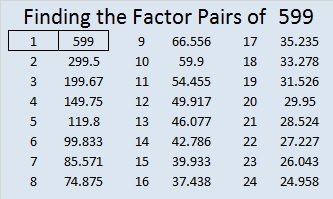How do we know that 599 is a prime number? If 599 were not a prime number, then it would be divisible by at least one prime number less than or equal to √599 ≈ 24.5. Since 599 cannot be divided evenly by 2, 3, 5, 7, 11, 13, 17, 19, or 23, we know that 599 is a prime number.

598 and Level 6

598 is the sum of all the prime number from 17 to 71.

598 is also the hypotenuse of the Pythagorean triple 230-552-598. What is the greatest common factor of those three numbers?

Here’s another easy as 1-2-3 number fact from Stetson.edu: (5^1) + (9^2) +(8^3) = 598.

Since 23 + 3 = 26 and 23 x 26 = 598, we know we are only 2 away from 24 x 25 = 600.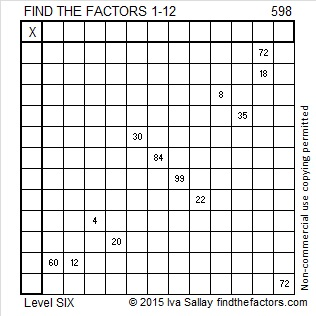Print the puzzles or type the solution on this excel file: 12 Factors 2015-08-24

—————————————————————————————————

• 598 is a composite number.
• Prime factorization: 598 = 2 x 13 x 23
• The exponents in the prime factorization are 1, 1, and 1. Adding one to each and multiplying we get (1 + 1)(1 + 1)(1 + 1) = 2 x 2 x 2 = 8. Therefore 598 has exactly 8 factors.
• Factors of 598: 1, 2, 13, 23, 26, 46, 299, 598
• Factor pairs: 598 = 1 x 598, 2 x 299, 13 x 46, or 23 x 26
• 598 has no square factors that allow its square root to be simplified. √598 ≈ 24.4540385.—————————————————————————————————594 and Level 2

It is as easy as 1-2-3 to remember this number fact from Stetson.edu:

(1^5) + (2^9) + (3^4) = 594

594 is the sum of the ten prime numbers from 41 to 79.

594 is even so it is divisible by 2.

5 + 9 + 4 = 18 so 594 is divisible by both 3 and 9 (and by 6 and 18 because 594 is even)

5 – 9 + 4 = 0 so 594 is divisible by 11.Print the puzzles or type the solution on this excel file: 12 Factors 2015-08-24

—————————————————————————————————

• 594 is a composite number.
• Prime factorization: 594 = 2 x 3 x 3 x 3 x 11, which can be written 594 = 2 x (3^3) x 11
• The exponents in the prime factorization are 1, 3, and 1. Adding one to each and multiplying we get (1 + 1)(3 + 1)(1 + 1) = 2 x 4 x 2 = 16. Therefore 594 has exactly 16 factors.
• Factors of 594: 1, 2, 3, 6, 9, 11, 18, 22, 27, 33, 54, 66, 99, 198, 297, 594
• Factor pairs: 594 = 1 x 594, 2 x 297, 3 x 198, 6 x 99, 9 x 66, 11 x 54, 18 x 33, or 22 x 27
• Taking the factor pair with the largest square number factor, we get √594 = (√9)(√66) = 3√66 ≈ 24.372115—————————————————————————————————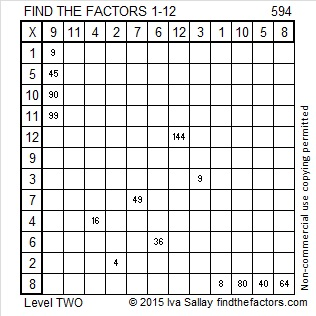586 and Level 1

586 is the hypotenuse of the Pythagorean triple 136-570-586. Which factor of 586 is the greatest common factor of those three numbers?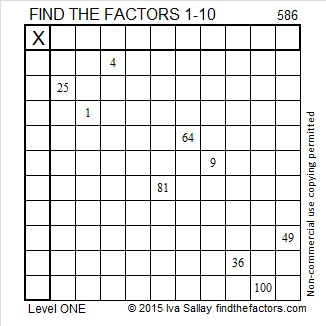Print the puzzles or type the solution on this excel file: 10 Factors 2015-08-17

—————————————————————————————————

• 586 is a composite number.
• Prime factorization: 586 = 2 x 293
• The exponents in the prime factorization are 1 and 1. Adding one to each and multiplying we get (1 + 1)(1 + 1) = 2 x 2 = 4. Therefore 586 has exactly 4 factors.
• Factors of 586: 1, 2, 293, 586
• Factor pairs: 586 = 1 x 586 or 2 x 293
• 586 has no square factors that allow its square root to be simplified. √586 ≈ 24.2074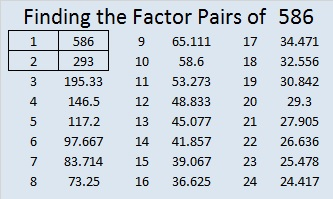—————————————————————————————————572 and Level 1

72 can be evenly divided by 4 so 572 is divisible by 4.

5 – 7 + 2 = 0, which can be evenly divided by 11, so 11 is a factor of 572.

572 is the hypotenuse of the Pythagorean triple 220-528-572. Which factor of 572 is the greatest common factor of those three numbers?Print the puzzles or type the solution on this excel file: 10 Factors 2015-08-03

—————————————————————————————————

• 572 is a composite number.
• Prime factorization: 572 = 2 x 2 x 11 x 13, which can be written 572 = (2^2) x 11 x 13
• The exponents in the prime factorization are 2, 1, and 1. Adding one to each and multiplying we get (2 + 1)(1 + 1)(1 + 1) = 3 x 2 x 2 = 12. Therefore 572 has exactly 12 factors.
• Factors of 572: 1, 2, 4, 11, 13, 22, 26, 44, 52, 143, 286, 572
• Factor pairs: 572 = 1 x 572, 2 x 286, 4 x 143, 11 x 52, 13 x 44, or 22 x 26
• Taking the factor pair with the largest square number factor, we get √572 = (√4)(√143) = 2√143 ≈ 23.91652—————————————————————————————————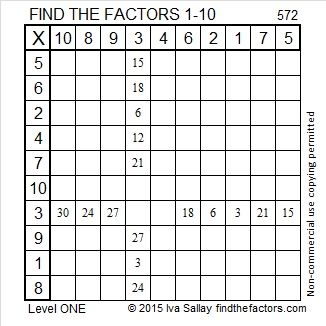564 and Level 6

Consecutive primes 281 and 283 add up to 564.

564 is made from three consecutive numbers so it can be evenly divided by 3. If the middle number is divisible by 3, then a number made from three consecutive numbers will also be divisible by 9. Is 564 divisible by 9? Why or why not?Print the puzzles or type the solution on this excel file: 10 Factors 2015-07-20

—————————————————————————————————

• 564 is a composite number.
• Prime factorization: 564 = 2 x 2 x 3 x 47, which can be written 564 = (2^2) x 3 x 47
• The exponents in the prime factorization are 2, 1, and 1. Adding one to each and multiplying we get (2 + 1)(1 + 1)(1 + 1) = 3 x 2 x 2 = 12. Therefore 564 has exactly 12 factors.
• Factors of 564: 1, 2, 3, 4, 6, 12, 47, 94, 141, 188, 282, 564
• Factor pairs: 564 = 1 x 564, 2 x 282, 3 x 188, 4 x 141, 6 x 94, or 12 x 47
• Taking the factor pair with the largest square number factor, we get √564 = (√4)(√141) = 2√141 ≈ 23.74868—————————————————————————————————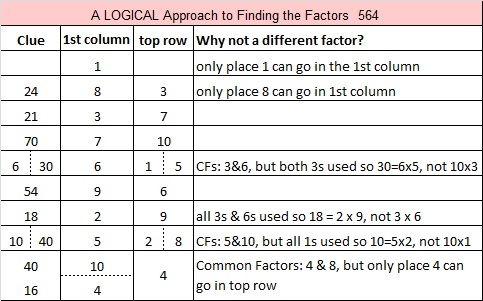562 and Level 4

562 is the sum of all the prime numbers from 23 to 71.

562 is also the hypotenuse of the Pythagorean triple 320-462-562. Which factor of 562 is the greatest common factor of those three numbers?Print the puzzles or type the solution on this excel file: 10 Factors 2015-07-20

—————————————————————————————————

• 562 is a composite number.
• Prime factorization: 562 = 2 x 281
• The exponents in the prime factorization are 1 and 1. Adding one to each and multiplying we get (1 + 1)(1 + 1) = 2 x 2 = 4. Therefore 562 has exactly 4 factors.
• Factors of 562: 1, 2, 281, 562
• Factor pairs: 562 = 1 x 562 or 2 x 281
• 562 has no square factors that allow its square root to be simplified. √562 ≈ 23.7065—————————————————————————————————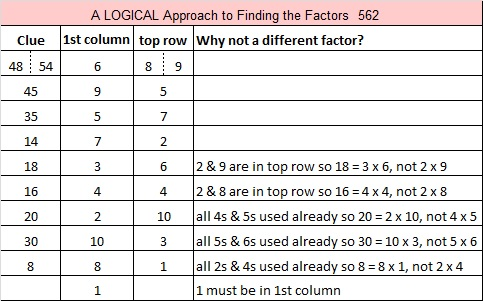560 and Level 3

560 is the hypotenuse of the Pythagorean triple 336-448-560. What is the greatest common factor of those three numbers?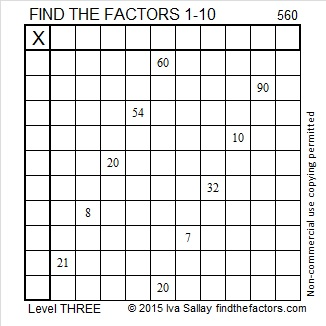Print the puzzles or type the solution on this excel file: 10 Factors 2015-07-20

—————————————————————————————————

• 560 is a composite number.
• Prime factorization: 560 = 2 x 2 x 2 x 2 x 5 x 7, which can be written 560 = (2^4) x 5 x 7
• The exponents in the prime factorization are 4, 1 and 1. Adding one to each and multiplying we get (4 + 1)(1 + 1)(1 + 1) = 5 x 2 x 2 = 20. Therefore 560 has exactly 20 factors.
• Factors of 560: 1, 2, 4, 5, 7, 8, 10, 14, 16, 20, 28, 35, 40, 56, 70, 80, 112, 140, 280, 560
• Factor pairs: 560 = 1 x 560, 2 x 280, 4 x 140, 5 x 112, 7 x 80, 8 x 70, 10 x 56, 14 x 40, 16 x 35 or 20 x 28
• Taking the factor pair with the largest square number factor, we get √560 = (√16)(√35) = 4√35 ≈ 23.664319—————————————————————————————————

A Logical Approach to solve a FIND THE FACTORS puzzle: Find the column or row with two clues and find their common factor. Write the corresponding factors in the factor column (1st column) and factor row (top row).  Because this is a level three puzzle, you have now written a factor at the top of the factor column. Continue to work from the top of the factor column to the bottom, finding factors and filling in the factor column and the factor row one cell at a time as you go.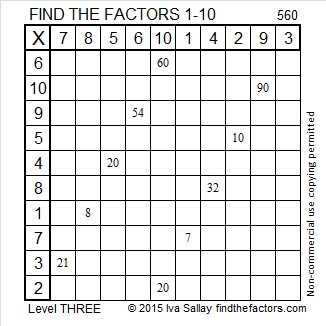559 and Level 2

All the prime numbers from 67 to 97 add up to 559, and all the prime numbers from 103 to 127 also add up to 559.

559 is also the hypotenuse of Pythagorean triple 215-516-559. What is the greatest common factor of those three numbers?Print the puzzles or type the solution on this excel file: 10 Factors 2015-07-20

—————————————————————————————————

• 559 is a composite number.
• Prime factorization: 559 = 13 x 43
• The exponents in the prime factorization are 1 and 1. Adding one to each and multiplying we get (1 + 1)(1 + 1) = 2 x 2 = 4. Therefore 559 has exactly 4 factors.
• Factors of 559: 1, 13, 43, 559
• Factor pairs: 559 = 1 x 559 or 13 x 43
• 559 has no square factors that allow its square root to be simplified. √559 ≈ 23.64318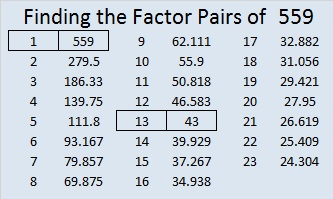—————————————————————————————————